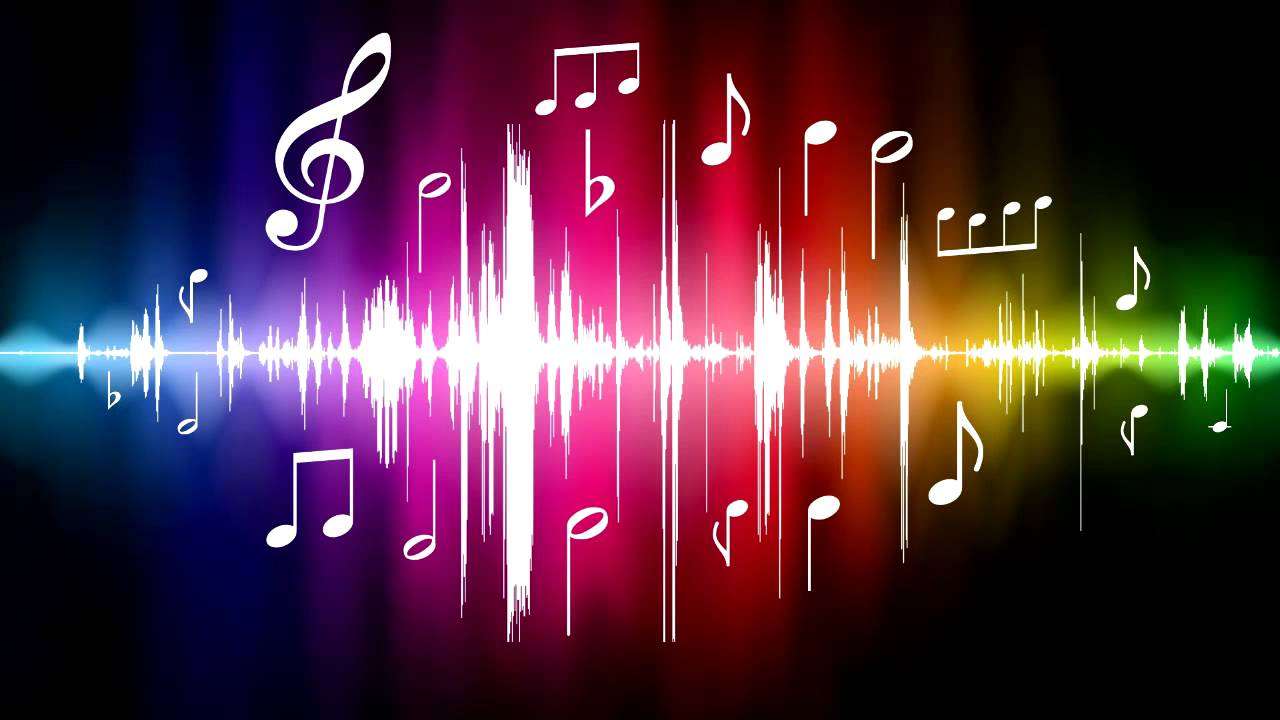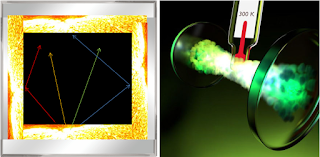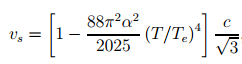Monday, 9 May 2016

The Speed of Sound in Light

This post will discuss something that I think is interesting, and wanted to think more about: the propagation of sound through light. One can show that the speed of sound in light is 57% the speed of light. I will talk about what the hell that actually means, and where that number comes from.Sound in light?

Sound in light? Huh?

The first thing one must wrap their head around is: what does it mean for sound to propagate through light? I devised a simple thought experiment to help me understand that.
Imagine two mirrors with springs on their backs, facing each other with light propagating between them. You can either imagine this as photons bouncing back and forth, or a standing electromagnetic wave. The mirrors are in mechanical equilibrium: the radiation pressure from the light reflecting off them is balanced by compression of the springs holding the mirrors in place.Two mirrors with a standing electromagnetic wave between them. Springs balance the radiation pressure, but if one mirror is moved, the induced Doppler shift will increase the radiation pressure on the opposite mirror, causing that spring to compress. This is my first foray into hand-drawn diagrams...sorry if it's terrible.
Now imagine you boop one of the mirrors, pushing it towards the other one. As light reflects off this mirror, it will be blue-shifted from the perspective of the other mirror. This blue-shifting will increase the magnitude of the radiation pressure, pushing the mirror back and compressing the spring a bit more. In a sense, pushing the first mirror caused an acoustic signal to propagate through the light to the other. The mirrors are both now in simple harmonic motion, coupled by the transfer of radiation overpressure and underpressure through blueshifting and redshifting as the mirrors move.

This scenario can be extended further, to an array of two-sided mirrors in an optical cavity, springs only on the mirrors on the end. Booping one end mirror would cause this blueshift-induced radiation overpressure to propagate down the line of mirrors. As the last mirror compresses the spring and rebounds, the wave will reverse direction.

The way I am picturing this scenario, the signal propagates through the light at the speed of light: as soon as you blueshift the first photon, it's already heading towards the other mirror at the speed of light.

The speed of sound in a photon gas

The scenario above envisioned light in mechanical equilibrium with mirrors. But what if it is also in thermal equilibrium? Now in this thought experiment, we have a box with mirrors on the inside, that is really hot (students of physics may recognize this as the canonical example of a blackbody). The mirrors are so hot that they start emitting blackbody radiation, which is reflected (or absorbed and re-emitted, depending on how you think about it) by the other mirrors in the box. Now what happens if you push on one side of the box?Left. A really crappy depiction I made of a photon gas: a mirrored box with really hot walls, with radiation bouncing around in the box. Right. The best online depiction I found on google images, from photonics.com.

The way I am picturing the situation, basically the same thing happens but not deterministically: the blackbody radiation from the mirror you push on gets blueshifted, increasing the radiation pressure felt on the opposite side. However, the radiation is emitted isotropically from the surface, and some of it goes off on an angle and reaches the side walls, and the radiation pressure excess from the boop gradually propagates towards the other side. So instead of just one c-retarded propagation from side to the other, there is a distribution in the arrival time of the mechanical information. I suppose the same thing happens when we push on one side of a box full of gas, and because there are so many gas molecules, the sound propagation is effectively deterministic.

At this point I have exhausted my ability to picture what would happen, and will examine a more rigorous argument. The speed of sound in a photon gas can be derived from its thermodynamic equation of state and associated equipartition theorem, which is basically similar to the ideal gas law, except it is derived by assuming that all the particles are going so fast that they are effectively massless (in that the lion's share of their energy is kinetic). The main difference between the regular and relativistic results is that for slow particles like atoms in a gas, the equipartition tells us that the average kinetic energy is 3/2 kT per atom, whereas in the relativistic case is is 3kT. This is basically because you're writing down the energy as pc instead of 1/2mv$^{2}$=$\frac{p^2}{2m}$, so there's no factor of two. There are some more involved derivations to get the number of photons and calculate the pressure based on the temperature, which essentially involve integrating the Planck spectrum over all frequencies (similar to the derivation of the Stefan-Boltzmann law). This is not necessary here.

To find the speed of sound in a gas we want the ratio of pressure to density:

$v^{2}=\frac{P}{\rho}$.

And from the photon gas equation of state we have a relationship between pressure and internal energy.

U=3PV

With a bit of relativity we can relate the regular density to the energy density by dividing it by $c^2$:

$\rho=\frac{1}{c^2}\frac{U}{V}=\frac{3P}{c^2}$

Plugging this back into the equation or the velocity, we have:

$v^{2}=P\frac{c^2}{3P}=\frac{c^2}{3}$.

Thus, we find that the speed of sound through a photon gas is one-over-root-three, or 57%, the speed of light. Notice that the factor of three comes from the number of dimensions used in the equipartition theorem. In one dimension, which my initial mirror scenario took place in, the speed of sound in light is the same as the speed of light itself.

Photon-Photon Interactions

In the above discussions, the "sound waves" are essentially just signals of increased radiation pressure that are transmitted through Doppler shifts. However, at high-enough energy densities, you can start to have the light interacting with itself, via electron-positron pairs that are produced from the vacuum. This self-interaction of light causes a slight slowdown in the speed. It is derived from quantum electrodynamics by Partovi here, and he derives a correction to the speed that depends on the fourth power of the ratio of the temperature of the blackbody to the temperature-equivalent of the electron mass, 5.9 gigakelvin (511 keV divided by Boltzmann's constant). The correction to the velocity is of order 10$^-5$ even at gigakelvin temperatures:This equation copied from Partovi's paper.
With this in mind, I can picture poking one side of a hot mirror box and expecting a small delay before the other side of the hot mirror box gets jolted.

The Relativistic Sound Speed in Astrophysics

This idea is neat, but is it important? This photon gas sound speed comes up in a few other places. The main one is baryon acoustic oscillations, sound waves through the very early universe that dictated the large-scale structure of the cosmos as the universe cooled down, still present in the periodic distribution of galaxy clusters. The very early universe was very hot, so there was a high energy photon gas just from thermal equilibrium, and all the massive particles zipzapping around were also highly relativistic. Thus, the speed associated with these baryon acoustic oscillations is that relativistic 0.57c.A telescope attempting to measure Baryon Acoustic Oscillations, from BNL.gov

Another question that arises is whether this is the absolute maximum for sound speed propagation. As I demonstrated in my thought experiment, one-dimensional coherent systems can exceed it, but what about in thermodynamic systems? A paper in Physical Review Letters last year argues that certain models of  neutron star interiors may predict sound speeds that exceed this limit. There is a lot that is unknown about neutron star interiors, and many models put constraints on the maximum and minimum sizes of neutron stars. These models then get selectively ruled out by newer neutron star discoveries. Bedaque and Steiner argue that given the available models and certain observations of particularly small neutron stars, the speed of sound inside some neutron stars must exceed 0.57c. Unfortunately I don't really understand enough of their analysis to say anything for insightful. Alternately, if this 0.57c is the true upper limit, it will rule out a lot of these models.

To summarize, I think that the idea of a speed of sound in light is a cool idea (this is how most of my blog posts end). I make it make sense to me using a thought experiment involving radiation pressure and Doppler shifting. For a more complete understanding, we go to photon gas thermodynamics and QED. There is one point where my research experience touches upon this concept: my undergraduate thesis in general relativity, the first independent physics research I conducted, involved finding fluid mass distributions that solve Einstein's equations. These mass distributions then had to satisfy certain conditions to make them physically realistic: mass couldn't be negative, density had to always decrease with radius, I wanted to avoid singularities, etc, but I also required that the speed of sound not exceed the speed of light. This extended the previous work of my supervisor Kayll Lake, who showed that many existing solutions to Einstein's equations violated these conditions. I wonder if anything would change if we demanded that v<0.57 c instead of v<c.

1.Nice description. Thanks!

2.Sound, which is a wave, which is motion cannot propagate through light which is also a wave which is also motion.

Sound is motion through baryonic matter whereas light is motion through Aetheric matter. There are two fundamental phenomena in the universe; Matter and Motion.

There is no such thing as time. Time IS Motion.

Nice article though. I enjoyed it.